# CSVTU Exam Papers – BE I Year – Engineering Mechanics– 2005

BE (1st Year)

Examination-2005

Engineering Mechanics

UNIT- I

Q.1 Attempt any two questions :

(a) A collar which may slide on a vertical rod is subjected to the three forces shown Determine .

(i) The voltage of the angle a for which the resultant of the three forces is horizontal,

(ii) The corresponding magnitude of the resultant.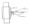(b) The three forces shown and a couple M are applied to angle bracket find the moment of the couple of the line action of the

resultant to the force system is to pass through

(i) point A

(ii) point B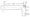(c) A slender rod of length L is loaded between peg C and vertical wail It supports a load P at end A. Neglecting fric and the weight

of the rod , determine the angle corresponding to equilibrium.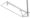UNIT- II

Q.2 Attempt any two questions :

(a)  A beam AB span 4 m is simply supported at the ends carries a concentrated load of 4 ken at distance of 1 from support A. It

also carries a uniformly distributed load 1.8kN/m up to a distance of 2.6 m from support A. Drav shear and bending moment

diagram for the beam AB.

(b) Using the method of joints , determine tie force in a member of the truss shown. State whether each member in tension or

compression.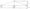(c) Determine the force in members DF, DE and CE of the shown.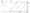UNIT- III

Q. 3 Answer any two questions :

(a) A 500-N force acts as shown on a 150 kg block placed on inclined plane . The coefficients of friction between the block and

the plane are μ s = 0.25 and μ k =.20.Determine whether the block is in equilibrium and find the value of the frict force.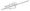(b) A 12° wedge is used to split a log. The coefficient of friction between the wedge and the wood is 0.40. Knowing that a force P

of magnitude 3.2 ken was required to insert the wedg determine the magnitude of the forces exerted on the log the wedge

after it has been inserted .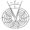(c) A flat belt is used to transmit a torque from pulley A to pulley B . The radius of each pulley is 50mm and the coefficient of

static friction is 0.30. The centre distance between the pulley is 200mm. Determine the largest torque which can be

transmitted of the allowable belt tension is 3 ken.UNIT- IV

Q.4 Attempt any two :

(a)Determine by direct integration the moment of inertia of the shaded area with respect to the y-axis.

(b)Determine the moment of inertia Ix and Icy of the area shown with respect to the censorial axes respectively parallel

and perpendicular to the side AB.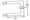(c) Determine the mass moment of inertia of a right circular cone with respect to an axis through the apex of the cone and

perpendicular to its longitudinal axis.

UNIT- V

Q.5 Attempt any two questions-:

(a) A cord is wrapped around a homogenous disk of radius r= 0.5 m and mass m = 15 kg . If the cord is pulled upward with a force

T of magnitude 180 N,

Determine :

(i) the acceleration of the center of the disk

(ii) the angular acceleration of the disk.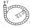(b) A sphere and a cylinder , each having the same mass the same radius are released from rest on an inclined. Determine

the velocity of each body after its has rolled through a distance corresponding to a change in elevatior h.

(c) A 2 kg sphere moving horizontally to the right with an initial velocity of 5 m/s strikes the lower the lower and B of an 8-k rigid

rod AB. The rod is suspended from a hinge at A an initially at rest. Knowing that the coefficient of restitution between the rod

and sphere is 0.80, determine the angular velocity of velocity of the rod and the velocity of the spherical immediately after

the impact.